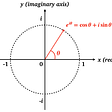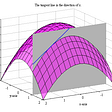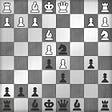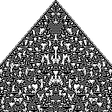# Types of models, algorithms, theories, and explanations

For the purposes of this post, a model is a nebulous concept related to a mathematical function, but not equivalent to it. A function is an “ideal” mathematical object, whereas a model can be limited to verifying that certain arguments correspond to a certain value in a function, but not telling how to generate the value from the arguments. Models could generate approximate results, that is, probability distributions instead of concrete values. Conversely, some models could correspond to functions that map into probability distributions but these models themselves generate only samples from these distributions.

I want to enumerate the types (or aspects) of models, which describe how models are described and executed (for either generation or verification).

Algorithms, theories, and explanations (in the sense that David Deutsch puts in this term in The Beginning of Infinity) are related to models and the classification which I come up with also applies to them.

## Stephen Wolfram’s general paradigms for theoretical science

Below, I’ll refer to these paradigms, so I include the picture for illustration:

## Types of models

The classification of models into types/aspects is fuzzy. For example, geometric models should perhaps be classified into “compartmentalisation/boundary” type if we look at the representation and into “analytical/equation-based” type if we look at these models from the perspective of analytic geometry.

• Compartmentalisation/boundary. Examples: maps, quadrant schemas. This type (along with the next one) roughly corresponds to Wolfram’s “structural” paradigm.
• Connectionistic/ontological. Examples: graphs, lists of things, ontologies, system/work/functional/etc. breakdown structures, reference frames, circuit diagrams, causal diagrams.
• Analytical/equation-based. Examples: many models of physics (classical mechanics, general relativity, thermodynamics, fluid mechanics, quantum mechanics), Structural Causal Models, Simulink models. This type corresponds to Wolfram’s “mathematical” paradigm.
• Algorithmic/rule-based (generation). Examples: imperative code, Knuth-style algorithms, cellular automata, tax law, rules of logic (including probabilistic logic!). This type corresponds to Wolfram’s “computational” paradigm.
• Principles/rules-based (verification only). Unlike all other types of models, this only permits verifying the results, but not producing them. Examples: David Deutsch’s Constructor theory, memory models of programming languages, traffic regulation, other codes of rules, code of regulation, codes of conduct, etiquette.
• Stochastic/randomised. Unlike most other types of models, stochastic models could only be used to generate results, but not to verify them. Examples: random walks, probability sampling.
• Statistical/vote-based/parallel. Examples: statistical classification, neural networks (both biological and artificial), biochemical computing (used by organisms without a nervous system), Monte Carlo simulation, swarm intelligence.
• Multicomputational: quantum algorithms

Real-life models often have distinct features or elements coming from different types. For example, Bayesian networks and Markov chains can be seen to have some features of ontological, analytical, algorithmic, and stochastic types of models. Evolutionary algorithms combine stochastic computation, rule-based verification/pruning, and statistical computation (of a whole population). Therefore, I also use the term “aspects” as a synonym for “types” to highlight that models could have multiple of them.

Would you classify models differently? Did I forget something?

This post was originally published on Substack.

--

--

--

## More from Engineering Ideas

Writings about software, systems, reliability, and data engineering, software operations, peopleware, philosophy, etc. by Roman Leventov. Originally published at https://engineeringideas.substack.com/

## e^(iπ) + 1 = 0: The Most Beautiful Theorem in Mathematics## Measuring Network Centrality## Permutations and Platonic Solids Hidden in the Slices of Hyper-Cubes## EURUSD Impulse Likely To End At 1.2268## Valuation — Time Value of Money (II)## How to Sacrifice in Chess## Bagging vs Boosting in Machine Learning training process## The Positive class? What should it be? in a machine learning binary classification problem?## The Role of Experimentation in Fundamental Machine Learning Research## Decoding Machine Learning